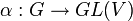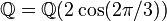# Linear representation of finite group over reals has invariant symmetric positive-definite bilinear form

## Statement

### For finite-dimensional vector space

Suppose$G$ is a finite group and$\alpha:G \to GL(V)$ (with$V$ finite-dimensional) is a linear representation of$G$ over a vector space$V$ over$\R$, the field of real numbers. Then, there exists a symmetric positive-definite bilinear form$b: V \times V \to V$ such that$\! b(\alpha(g)v,\alpha(g)w) = b(v,w) \ \forall \ g \in G, \ v,w \in V$

In other words, we can think of$b$ as a dot product invariant under the action of$G$.

Further, if we choose a basis for$V$ that is an orthonormal basis for$b$, then in this basis, all the matrices for$\alpha(g), g \in G$, are orthogonal matrices, i.e., as elements of$O(n,\R)$ where$n$ is the dimension of$V$ (and hence the degree of$\alpha$). Thus, another formulation is that every finite-dimensional linear representation over the real numbers is an orthogonalizable linear representation.

## Related facts

### Not true for all subfields of reals

It is possible to have a subfield$K$ of the real numbers with the property that a linear representation can be realized over that subfield, but it cannot be realized using orthogonal matrices of that subfield, because it does not possess an invariant inner product. Note that all examples of this kind must involve representations of degree more than one.

Here are some examples:

Representation Degree Group Linear representation theory of group Smallest subfield of reals where the representation is realized Degree of extension of rationals Smallest subfield of reals where the representation is realized using orthogonal matrices Degree of extension of rationals
standard representation of symmetric group:S3 2 symmetric group:S3 linear representation theory of symmetric group:S3$\mathbb{Q} = \mathbb{Q}(2\cos(2\pi/3))$ 1$\mathbb{Q}(\sqrt{3}) = \mathbb{Q}(\cos(2\pi/3),\sin(2\pi/3))$ (quadratic extension of$\mathbb{Q}$) 2
faithful irreducible representation of dihedral group:D10 2 dihedral group:D10 linear representation theory of dihedral group:D10$\mathbb{Q}(\sqrt{5}) = \mathbb{Q}(2\cos(2\pi/5))$ 2$\mathbb{Q}(\cos(2\pi/5),\sin(2\pi/5))$ 4